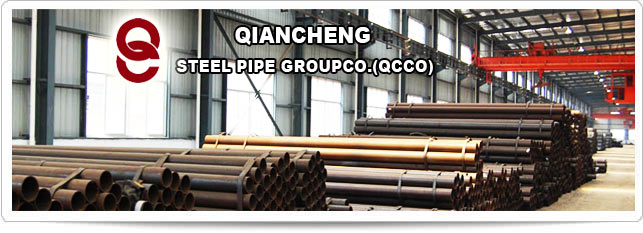# Steel Pipe Calculation Formulas

Home >One of the Top 500 enterprises in China foreign trade

QCCO was approved as a member of “China Association for Contracting Projects Abroad “and granted a membership certificate on Sep 28,2005; “Credibility Rating AAA certificate in Foreign Trade” was granted to QCCO by China Shippers’ Association## Steel Pipe Calculation Formulas

Steel Pipe Calculation Formulas

Fomulas to calculate area and weight of empty steel pipes and steel pipes filled with water

To calculate the cross section area of a steel pipe:

A = 0.785di2

where

A = cross-sectional area of pipe (Square Inches)

di = inside diameter (inches)

To calculate the weight of empty steel pipes:

wp = 10.6802 t (do - t)

where

wp =weight of steel pipe (Pounds per Foot Pipe)

t = pipe wall thickness (Inches)

do = outside diameter (inches)

To calculate the weight of water in pipes filled with water:

ww = 0.3405 di 2

where

ww = weight of steel pipe filled with water (Pounds per Foot Pipe)

di = inside diameter (inches)

To calculate the outside surface area of steel pipes:

Ao = 0.2618 do

where

Ao = outside area of pipe - per foot (Square Feet)

do = outside diameter (inches)

To calculate the inside surface area of steel pipes:

Ai = 0.2618 di

where

Ai = inside area of pipe - per foot (Square Feet)

di = inside diameter (inches)

To calculate the area of the metal:

Am = 0.785 (do 2 - di 2)

where

Am = area of the metal (Square inches)

di = inside diameter (inches)

do = outside diameter (inches)Quickly Find Products Information And Pricing